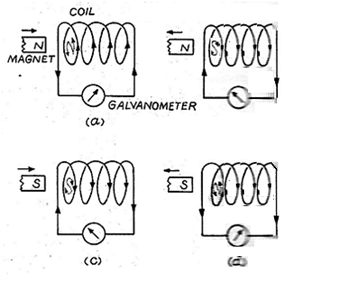# Explain Faraday's 1st and 2nd laws.

## Question:

Explain Faraday's 1st and 2nd laws.

## Electromagnetic Induction

Whenever magnetic flux associated with the coil or circuit changes, an e. m. f. or current is induced in the coil.This phenomenon is called as electromagnetic induction. If two coils are kept nearby and current in one coil is changed, an e.m. f. or current is induced in the second coil due to change in magnetic flux in the first coil.Experimental arrangement: :- A coil of conducting wire is connected to a sensitive galvanometer. A strong magnet is held near the coil as shown..

c) Procedure and observations:-

1) When the magnet is held stationary, the galvanometer doesn?t show any deflection.

2) When the magnet is moved towards the coil, suddenly the galvanometer shows momentary deflection.

3) When the magnet is moved suddenly away from the coil, the galvanometer shows deflection in opposite direction.

4) When the magnet is moved rapidly, greater deflection in the galvanometer is observed.

5) Similar results are obtained when the magnet is fixed and the coil is moved.

d) Explanation::-

1) Whenever there is relative motion between coil and the magnet, then magnetic field at every point inside the coil changes. Therefore, magnetic flux associated with the coil changes. This change in flux produces an induced e. m. f. or current in the coil.

2) When there is no relative motion between the coil and the magnet, there is no change in the magnetic flux linked with the coil. Due to this, no e. m. f. or current is induced in the coil.

3) Faster the relative motions between the coil and the magnet, faster the change in magnetic flux. Due to this, greater e. m. f. or current is induced in the coil.

1st law :- The flux through a coil or a circuit changes, an induced emf is set up in the coil or circuit whose magnitude is equal to the negative of rate of change of magnetic flux.

If {eq}\space \Delta \phi, \space \Delta t {/eq} are the changes in magnetic flux and in time

{eq}\frac{\Delta \phi }{\Delta t} {/eq} is the rate of change of magnetic flux

e = magnitude of the induced emf then e = {eq}-\frac{\Delta \phi}{\Delta t} {/eq}

As {eq}\Delta t \rightarrow 0, e = -\frac { \Delta \phi }{\Delta t} \rightarrow e = - \frac{ d \phi}{ d t } {/eq}

If the coil in the circuit has N turns then e = - N {eq}\frac { d \phi} { d t } {/eq}

ii) 2nd law( Lenz's law ) :- The direction of induced emf or current in any circuit or coil is such as to oppose the cause that produces it. This opposite direction is denoted by ?ve sign..